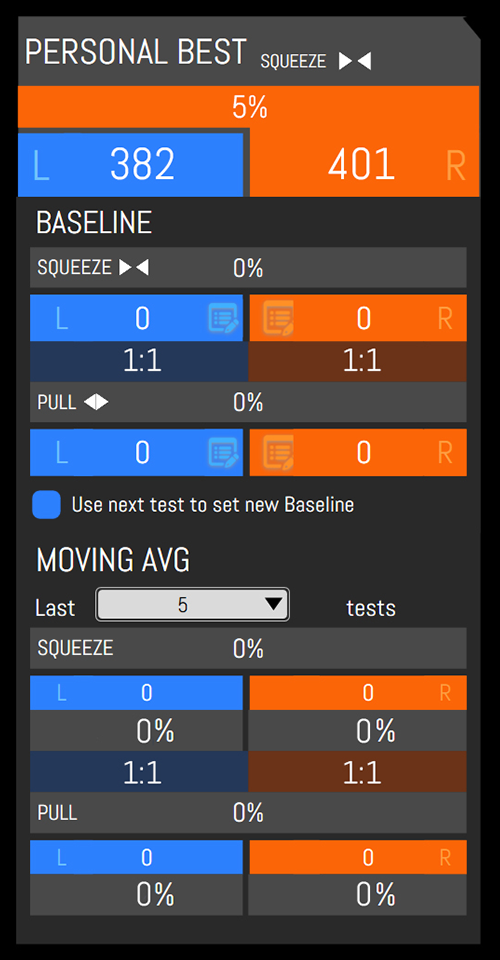# Understanding Results in the ForceFrame App

When you run a ForceFrame test in the ForceFrame App, a number of metrics are calculated automatically.  Here's a rundown of what the on-screen metrics mean.

Related:   Understanding NordBord Results in the Windows App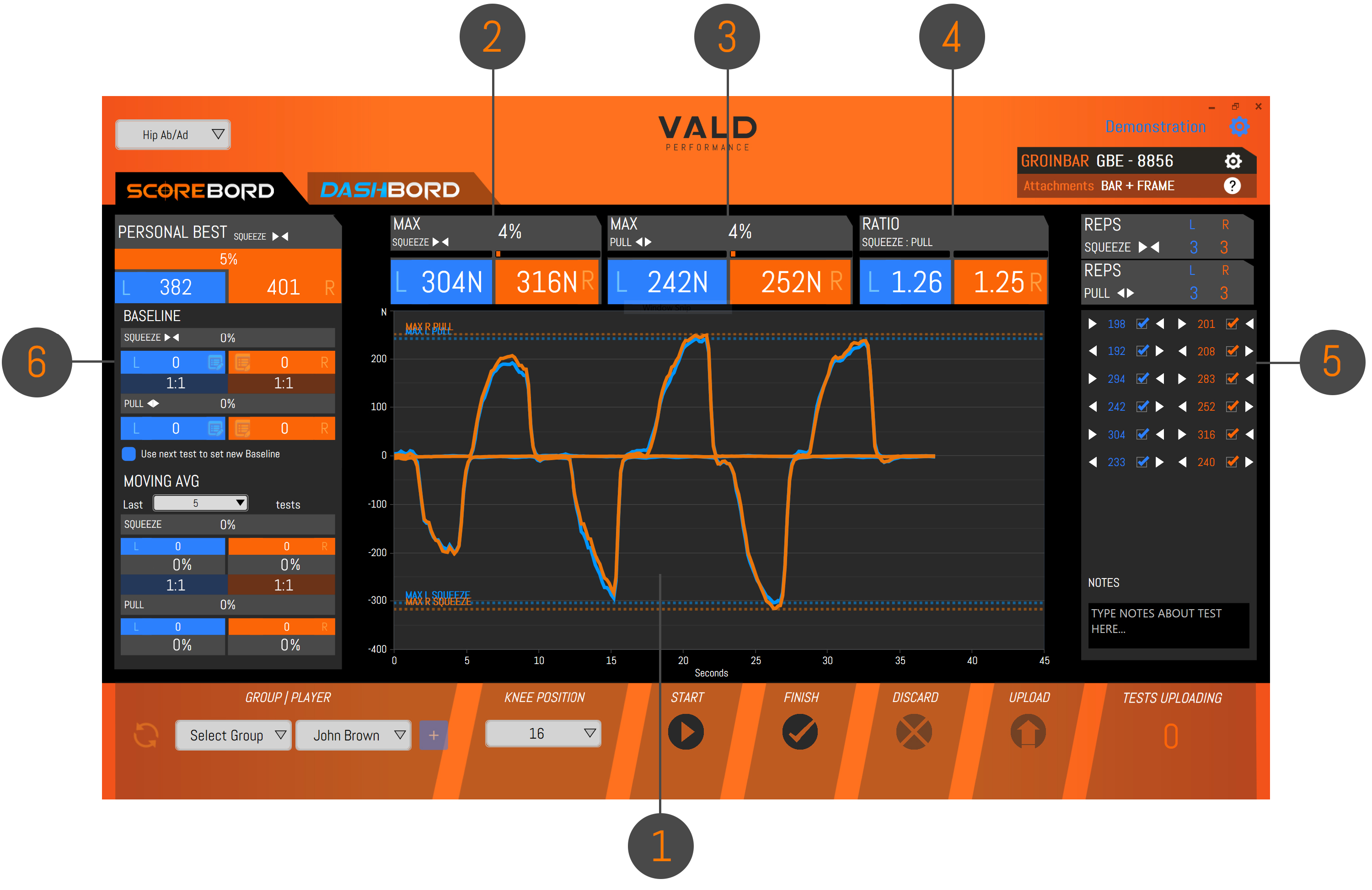1 The main graphing window shows a force-time trace during a test  Y-axis = force in newtons (N) X-axis = time in seconds (s) Blue line = left leg force Orange line = right leg force Negative values (<0N) = force on inner sensors Positive values (>0N) = force on outer sensors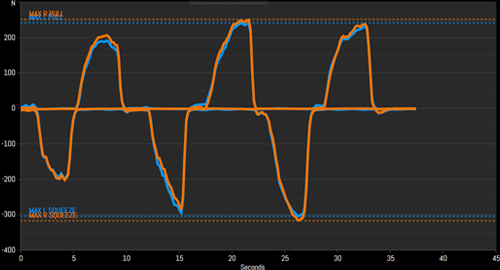2 The top-left results panel shows maximum "Squeeze" (i.e. inner sensor) results:  Maximum left leg force is shown in blue Maximum right leg force is shown in orange Imbalance (i.e. percentage difference between left and right maximums) is shown in grey Note: the text in brackets refers to the movement type being assessed (e.g. AD = adduction)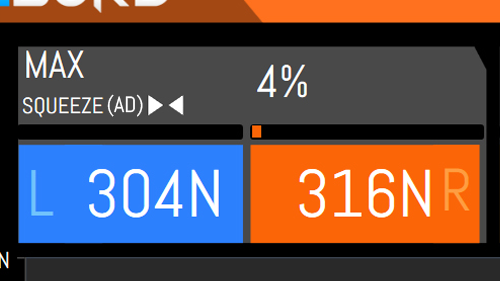3 The top-middle results panel shows maximum "Pull" (i.e. outer sensor) strength results:  Maximum left leg force is shown in blue Maximum right leg force is shown in orange Imbalance (i.e. percentage difference between left and right maximums) is shown in grey Note: the text in brackets refers to the movement type being assessed (e.g. AB = abduction)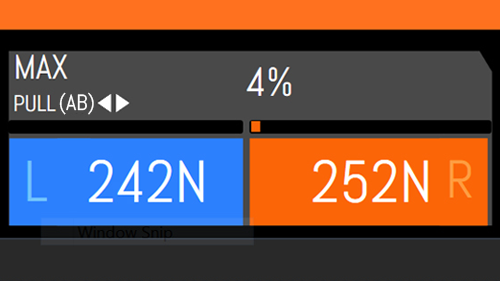4 The top-right results panel shows a ratio of maximum Squeeze force : maximum Pull force (i.e. >1:1 = Squeeze is dominant,  <1:1 = Pull is dominant)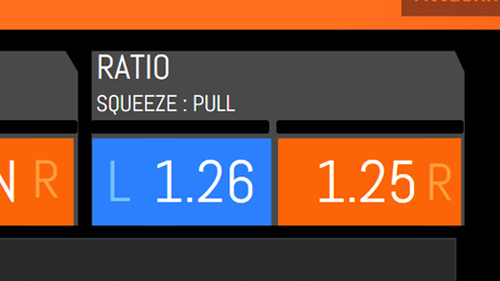5 The right-hand results bar shows rep-by-rep results for left and right leg.  Arrows indicate whether a rep is a Squeeze rep (inner sensors) or a Pull rep (outer sensors). Unwanted reps (e.g. accidental reps or reps performed with undesirable form) can be de-selected using the checkboxes next to each rep.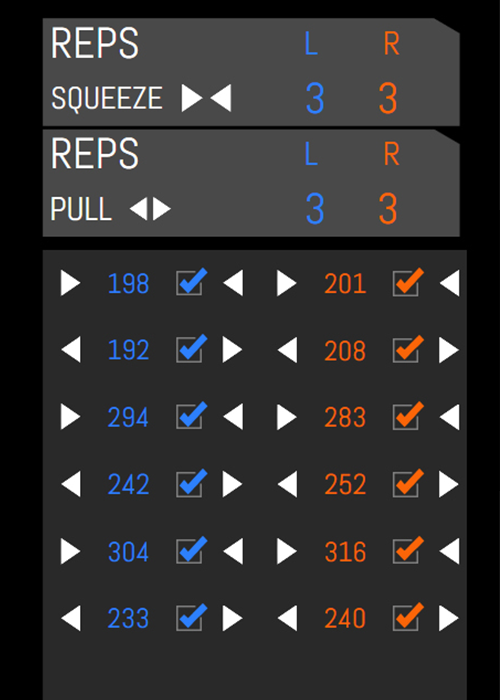6 The left-hand results bar shows reference points for the person being tested, in the test type being conducted:  Personal Best is their highest ever left and right leg results for that test Baseline is an editable reference point (often recorded at the start of a preseason or season) Moving Avg is the average result from the last 1-10 tests